# Equality of Series

## Homework Statement

Here is my equation that I want to find bs values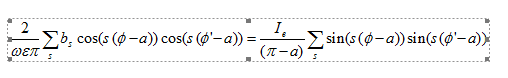## The Attempt at a Solution

I convert sin to cos.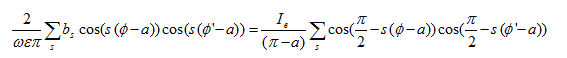for bs at s=0 I get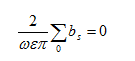and if s is not zero I can't derive a clear answer for bs.

but in electronic engineer book that I read it wrote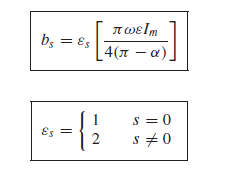Last edited:

Delta2
Homework Helper
Gold Member
My advice will be to use the trigonometric identities ##sin(A)sin(B)=\frac{1}{2}(cos(A-B)-cos(A+B))## and ##cos(A)cos(B)=\frac{1}{2}(cos(A-B)+cos(A+B)## for ##A=B=s(\phi-\alpha)##.

Thank you for your best suggestion
I did it for both side so I have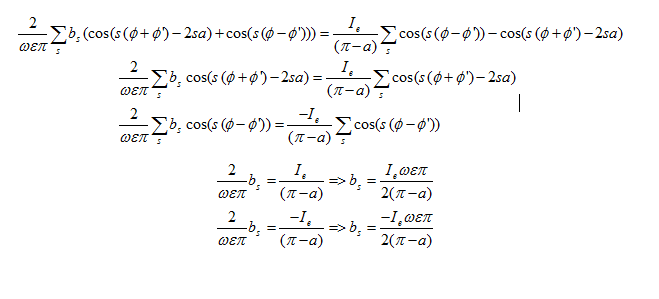but it doesn't the same or I can't derive book answer , what is my mistake?

Delta2
Homework Helper
Gold Member
Ok sorry my suggestion doesn't seem to work, more specifically when you move from line 1 to line 2 and 3 of your last post, that is sufficient but not necessary . Try instead to transfer everything to one side and use the identity ##CcosAcosB-CsinAsinB=Ccos(A+B)##

Sorry this doesn't seem to work either, I am all out of ideas for now.

Cant think of anything else than to expand any term of the form ##sin(s(\phi-\alpha))=sin(s\phi)cos(s\alpha)-sin(s\alpha)cos(s\phi)## and similar for cosines and see if you can get anything in the end.​

Last edited:
Ray Vickson
Homework Helper
Dearly Missed

## Homework Statement

Here is my equation that I want to find bs values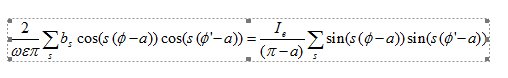## The Attempt at a Solution

I convert sin to cos.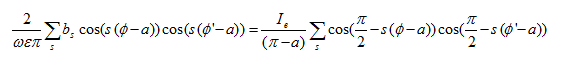for bs at s=0 I get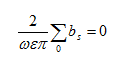and if s is not zero I can't derive a clear answer for bs.

but in electronic engineer book that I read it wroteYou need to tell us if the summation index ##s## goes over ##0,1,2, \ldots## or over ##\ldots -2, -1,0,1,2, \ldots## That can make a real difference.

•Delta2
Delta2
Homework Helper
Gold Member
Well seems to me the only way to make it work is s to take values in the whole integer set from negative to positive. Then what he did in post #3 is pretty much valid with some additional correction and justification regarding line 3 and 5 of post #3.

Ray Vickson
Homework Helper
Dearly Missed
Well seems to me the only way to make it work is s to take values in the whole integer set from negative to positive. Then what he did in post #3 is pretty much valid with some additional correction and justification regarding line 3 and 5 of post #3.

I was hoping to hear from the OP himself/herself.

•SammyS
Delta2
Homework Helper
Gold Member
I was hoping to hear from the OP himself/herself.

Well this thing puzzled me also, I thought my first suggestion should work because is the only straightforward way to transform different products (product of cosines and product of sines) to a sum of the same things. I don't know maybe I am still wrong and the solution is totally different, but seems to me its working (with some corrections) for s through all integers.

Thanks for you response.
m should be positive and m=0,1,2,3,....

SammyS
Staff Emeritus
Homework Helper
Gold Member
Thanks for you response.
m should be positive and m=0,1,2,3,....
Ray's question regarding indices was in regards to s, not m .

Last edited:
Ray Vickson
Homework Helper
Dearly Missed
Thanks for you response.
m should be positive and m=0,1,2,3,....

What is 'm'? Your original post had no 'm' in it anywhere. It did have an 's', though, and the issue is about the range of 's'. If that range is infinite (either one-way or two-way) the right-hand-side in your original equation is a divergent infinite series. So, I am not sure your question makes any mathematical sense.

Delta2
Homework Helper
Gold Member
However Baby1 if s is taking values in bounded integer sets of the form (-k,-k+1,...,-1,0,1,...,k-1,k) the solution can be ##b_s=\frac{I\omega\epsilon\pi}{2(\pi-\alpha)}## and satisfies both line 2 and line 3 of your post #3. For line 2 is obvious for line 3 you have to find out as I am sure you will .

Last edited:
•baby_1